Next: Estimating Mutation Matrices Up: Better Dayhoff Matrices Previous: The CreateDayMatrix Function

## Percent Identity and PAM Distance

Recall, a 1-PAM mutation matrix measures a unit of evolution where the expected change is exactly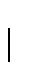of the amino acids. It is clear that a 2-PAM evolution will not produce a 2% change since some changes may mutate back to the original amino acid. (A 2-PAM evolution is equivalent to two separate 1-PAM evolutionary units.) The percentage identity, pi, is defined to be the number of identical bases between two sequences in an alignment. It is is related to the PAM distance, p, by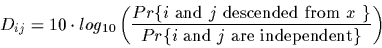In the above formula we need both the mutation matrix and the frequency vector. The frequency vector can be derived from any mutation matrix. Recall the symmetry relation:

fi Mji = fj Mij

Setting j=1 and rearranging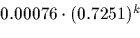On the other hand we know that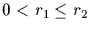, consequentlyThe formula for pi(p) can be made independent of the frequencies fi as:for any mutation matrix M, in particular for Mp which is also a mutation matrix. The following function computes the percent identity for a given PAM distance.
> PamToPerIdent := proc( pam:real, logPAM1:array(real,20,20) )
> description
>     'compute the percentage identity that a pam distance will leave';
> if pam < 0 then error('invalid percentage range') fi;
>
> num := den := 0;
> M := exp(pam*logPAM1);
> for i to 20 do
>     num := num + M[i,1]*M[i,i]/M[1,i];
>     den := den + M[i,1]/M[1,i]
>     od;
> 100*num/den;
> end:

By definition, the following should be 99%
> PamToPerIdent(1,logPAM1);
99.0000

> PamToPerIdent(250,logPAM1);
16.9051

the classical PAM value gives about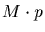identity. Many believe that approximately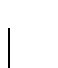identity is needed to detect homology based on sequence data alone.

Computing the inverse function, PerIdentToPam, is somewhat more difficult. Since it is very unlikely that a closed form solution exists, we use Newton's method for solving equations.

Newton's method begins with an initial guess x0 and computes x1,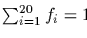using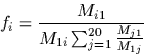where g(x) is the equation to be solved in the unknown x and gx'(x) is the derivative of g(x) with respect to x. In our case, the equation is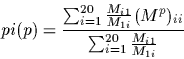and our unknown is p, the PAM distance. Computing the derivative of Mp with respect to pis not so complicated. Recall that M is a square matrix so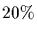is also a square matrix and using the series definition we can compute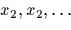(M andnecessarily commute under matrix multiplication). As expected the derivative of powers of matrices has the same expression as for scalars.

Consequently the derivative of the equation is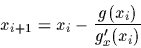This exercise also shows how to protect a Newton iteration from going astray by using binary search techniques. Newton's method may or may not converge on a solution. When it does, it does so quickly. However, often it does not converge at all.

> PerIdentToPam := proc( pi:real, logPAM1:array(real,20,20) )
> description 'compute the PAM distance which results in the given
>     percentage identity';

check the argument range and trivial case
> if pi <= 0 or pi > 100 then error('invalid percentage range') fi;
> if pi=100 then return(0) fi;

Compute the amino acid frequencies as described before
> AF := CreateArray(1..20);
> M := exp(logPAM1);
> for i to 20 do AF[i] := M[i,1]/M[1,i] od;
> AF := AF/sum(AF);

Now check that the percentage identity is not less than its asymptotic value. That is, for PAM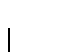, the percentage identity does not decrease to zero but to the percentage identity of two random sequences. This value is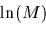> asy := AF*AF;
> if pi/100 <= asy then
>     error('pi cannot be less than the asymptotic value',100*asy) fi;
>

For reasons which escape the scope of this tutorial, the following is a good initial guess for the PAM value.
> pam := -100*ln(pi/100-asy);

Next, the PAM value will be bound below by lo and above by hi. Setting the above bound to 5 times the initial guess is sufficient.
> lo := 0;  hi := 5*pam;

We will now iterate until we reach the desired accuracy, i.e. the difference between the bounds is close to the machine epsilon.
> while hi-lo > hi*DBL_EPSILON*10 do
>    mp := exp(pam*logPAM1);
>    m1p := logPAM1*mp;
>    num := -pi/100;
>    den := 0;
>    for i to 20 do
>         num := num + AF[i]*mp[i,i];
>         den := den + AF[i]*m1p[i,i]
>         od;

Reset lo or hi as appropriate
>    if num >= 0 then lo := pam else hi := pam fi;
>    incr := -num/den;
>    pam := pam + incr;

If the increment squared is relatively less than the machine epsilon we can stop the iteration. Notice that when doing Newton's iteration, the relative error is proportional to the square of the previous relative error.
>    if abs(incr)^2 < abs(pam)*DBL_EPSILON then break fi;

If the value falls outside the bounds, then the Newton's value is not converging, so we just compute the midpoint of the bounds as the next guess.
>    if pam <= lo or pam >= hi then pam := (lo+hi)/2 fi;
>    od;
> pam
> end:

Again, by definition, the following should evaluate to 1.
> PerIdentToPam(99,logPAM1);
1.0000

A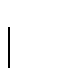identity corresponds to PAM 278.5236.
> PerIdentToPam(15,logPAM1);
278.5236Next: Estimating Mutation Matrices Up: Better Dayhoff Matrices Previous: The CreateDayMatrix Function
Gaston Gonnet
1998-09-15